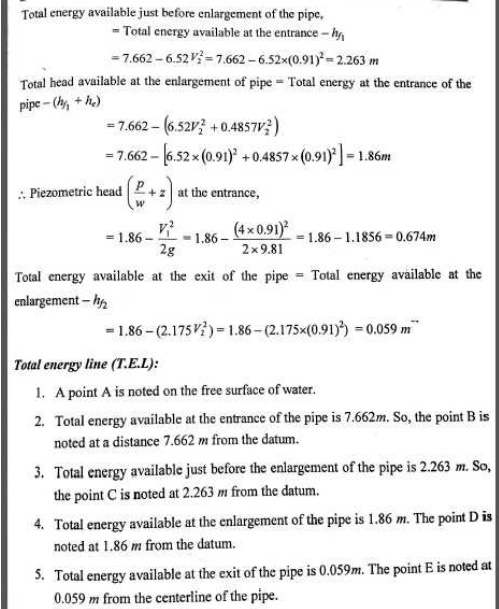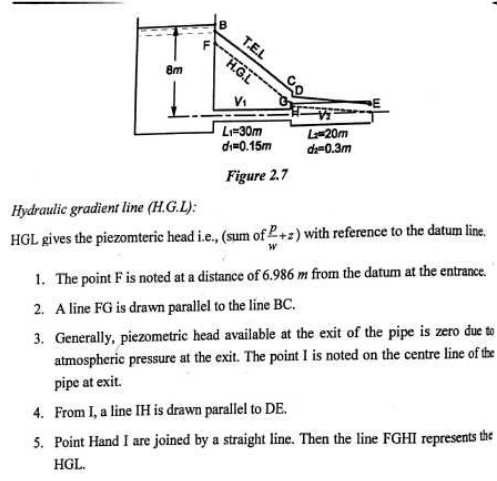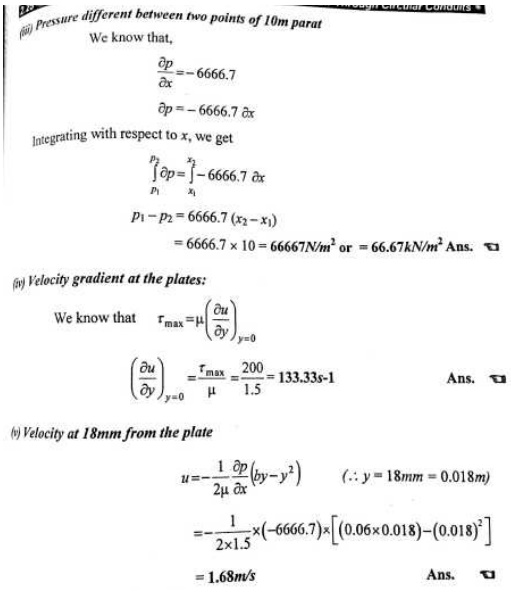Home | | Fluid Mechanics and Machinery | Important Answers and Solved Problems: Fluid Flow Through Circular Conduits

# Important Answers and Solved Problems: Fluid Flow Through Circular Conduits

Mechanical - Fluid Mechanics And Machinery - Flow Through Circular Conduits

FLOW THROUGH CIRCULAR CONDUITS

1. Define viscosity (u).

Viscosity is defined as the property of a fluid which offers resistance to the movement of one layer of fluid over another adjacent layer of the fluid.Viscosity is also defined as the shear stress required to produce unit rate of shear strain.

2. Define kinematic viscosity.

Kniematic viscosity is defined as the ratio between the dynamic viscosity and density of fluid. It is denoted by μ.

3. What is minor energy loss in pipes?

The loss of head or energy due to friction in a pipe is known as major loss while loss of energy due to change of velocity of fluid in magnitude or direction is called minor loss of energy. These include,

a. Loss of head due to sudden enlargement.

b. Loss of head due to sudden contraction.

c. Loss of head at entrance to a pipe.

d. Loss of head at exit of a pipe.

e. Loss of head due to an obstruction in a pipe.

f. Loss of head due to bend in a pipe.

g. Loss of head in various pipe fittings.

4. What is total energy line?

Total energy line is defined as the line which gives the sum of pressure head, datum head and kinetic head of a flowing fluid in a pipe with respect to some reference line. It is also defined as the line which is obtained by joining the tops of all vertical ordinates showing sum of the pressure head and kinetic head from the centre of the pipe.

5. What is hydraulic gradient line?

Hydraulic gradient line gives the sum of (p/w+z) with reference to datum line. Hence hydraulic gradient line is obtained by subtracting v2 / 2g from total energy line.

6. What is meant by pipes in series?

When pipes of different lengths and different diameters are connected end to end, pipes are called in series or compound pipe. The rate of flow through each pipe connected in series is same.

7. What is meant by pipes in parallel?

When the pipes are connected in parallel, the loss of head in each pipe is same. The rate of flow in main pipe is equal to the sum of rate of flow in each pipe, connected in parallel.

8. What is boundary layer and boundary layer theory?

When a solid body immersed in the flowing fluid, the variation of velocity from zero to free stream velocity in the direction normal to boundary takes place in a narrow region in the vicinity of solid boundary. This narrow region of fluid is called boundary layer. The theory dealing with boundary layer flow is called boundary layer theory.

9. What is turbulent boundary layer?

If the length of the plate is more then the distance x, the thickness of boundary layer will go on increasing in the downstream direction. Then laminar boundary becomes unstable and motion of fluid within it, is disturbed and irregular which leads to a transition from laminar to turbulent boundary layer.

10. What is boundary layer thickness?

Boundary layer thickness (S) is defined as the distance from boundary of the solid body measured in y-direction to the point where the velocity of fluid is approximately equal to 0.99 times the free steam (v) velocity of fluid.

11. Define displacement thickness

Displacement thickness (S*) is defined as the distances, measured perpendicular to the boundary of the solid body, by which the boundary should be displaced to compensate for the reduction inflow rate on account of boundary layer formation.

12. What is momentum thickness?

Momentum thickness (0) is defined as the distance, measured perpendicular to the boundary of the solid body, by which the boundary should be displaced to compensate for the reduction in momentum of flowing fluid on account of boundary layer formation.

13.Mention the general characteristics of laminar flow.

There is a shear stress between fluid layers

No slip’ at the boundary

The flow is rotational

There is a continuous dissipation of energy due to viscous shear

14.  What is Hagen poiseuille’s formula ?

P1-P2 / pg = h f = 32 µ UL / _gD2

The expression is known as Hagen poiseuille formula .

Where P1-P2 / _g = Loss of pressure head U = Average velocity

µ = Coefficient of viscosity                        D = Diameter of pipe

L = Length of pipe

15.What are the factors influencing the frictional loss in pipe flow ?

Frictional resistance for the turbulent flow is

i. Proportional to vn where v varies from 1.5 to 2.0 . ii. Proportional to the density of fluid .

iii. Proportional to the area of surface in contact . iv. Independent of pressure .

v. Depend on the nature of the surface in contact .

16.  What is the expression for head loss due to friction in Darcy formula ?

hf = 4fLV2 / 2gD

Where        f = Coefficient of friction in pipe        L = Length of the pipe

D = Diameter of pipe              V = velocity of the fluid

17.            What do you understand by the terms

a) major energy losses , b) minor energy losses Major energy losses : -

This loss due to friction and it is calculated by Darcy weis bach formula and chezys formula .

Minor energy losses :- This is due to

h.     Sudden expansion in pipe .ii. Sudden contraction in pipe . iii. Bend in pipe .iv. Due to obstruction in pipe .

18. Give an expression for loss of head due to sudden enlargement of the pipe :

he = (V1-V2)2 /2g

Wherehe = Loss of head due to sudden enlargement of pipe . V1 = Velocity of flow at section 1-1

V2 = Velocity of flow at section 2-2

19.Give an expression for loss of head due to sudden contraction : hc =0.5 V2/2g

Where hc = Loss of head due to sudden contraction . V = Velocity at outlet of pipe.

20. Give an expression for loss of head at the entrance of the pipe hi =0.5V2/2g

where hi = Loss of head at entrance of pipe .

V = Velocity of liquid at inlet and outlet of the pipe .

21. What is sypon ? Where it is used: _

Sypon is along bend pipe which is used to transfer liquid from a reservoir at a higher elevation to another reservoir at a lower level .

Uses of sypon : -

1. To carry water from one reservoir to another reservoir separated by a hill ridge .

2. To empty a channel not provided with any outlet sluice .Study Material, Lecturing Notes, Assignment, Reference, Wiki description explanation, brief detail
Mechanical : Fluid Mechanics And Machinery : Flow Through Circular Conduits : Important Answers and Solved Problems: Fluid Flow Through Circular Conduits |